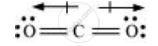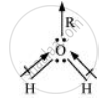# Although Both Co2 and H2o Are Triatomic Molecules, the Shape of H2o Molecule is Bent While that of Co2 is Linear. Explain this on the Basis of Dipole Moment. - Chemistry

Although both CO2 and H2O are triatomic molecules, the shape of H2O molecule is bent while that of CO2 is linear. Explain this on the basis of dipole moment.

#### Solution 1

In CO2, there are two C=O bonds. Each C=O bond is a polar bond.

The net dipole moment of CO2 molecule is zero. This is possible only if CO2 is a linear molecule. (O=C=O). The bond dipoles of two C=O bonds cancel the moment of each other.

Whereas, H2O molecule has a net dipole moment (1.84 D). H2O molecule has a bent structure because here the O—H bonds are oriented at an angle of 104.5° and do not cancel the bond moments of each other.

#### Solution 2

According to experimental results, the dipole moment of carbon dioxide is zero. This is possible only if the molecule is linear so that the dipole moments of C–O bonds are equal and opposite to nullify each other.Resultant μ = 0 D

H2O, on the other hand, has a dipole moment value of 1.84 D (though it is a triatomic molecule as CO2). The value of the dipole moment suggests that the structure of H2O molecule is bent where the dipole moment of O–H bonds are unequal.Concept: Bond Parameters - Polarity of Bonds
Is there an error in this question or solution?

#### APPEARS IN

NCERT Chemistry Part 1 and 2 Class 11
Chapter 4 Chemical Bonding and Molecular Structure
EXERCISES | Q 4.15 | Page 134